Courses
Courses for Kids
Free study material
Offline Centres
More

# NCERT Solutions for Class 12 Maths Chapter 12 - Linear ProgrammingLast updated date: 05th Dec 2023
Total views: 475.5k
Views today: 13.75k

## NCERT Solutions for Class 12 Maths Chapter 12 - Linear Programming - Free PDF Download

Class 12 Maths Chapter 12 explains the various concepts and principles of linear programming. Students will learn these concepts and solve the problems given in the exercise to build their concepts well. To make this process easier, download and refer to the CBSE Class 12 Maths Chapter 12 Linear Programming NCERT Solutions developed by the experts. Learn how to attempt problems related to linear programming and develop your answering skills.

NCERT Solutions for Class Maths Chapter-12 PDF is available for you to download and gain more knowledge about the subject. You can visit the official website of Vedantu to download this PDF file and make the most out of it. The Linear Programming Class 12 NCERT PDF has solutions related to the chapter prepared by the experts. If you are looking for the necessary solutions for Class 12 Maths Chapter 12, you are in the right place. The Linear programming Class 12 NCERT Solutions will provide you with more insights into the chapter in detail and help you understand it at an advanced level. You can get in touch with our experts who have prepared the NCERT solutions for Class 12 Maths Chapter 12 PDF file to clear your doubts.

## Linear Programming Chapter at a Glance - Class 12 NCERT Solutions

• A linear programming problem is one that is concerned with finding the optimal value (maximum or minimum) of a linear function of several variables (called objective function) subject to the conditions that the variables are nonnegative and satisfy a set of linear inequalities (called linear constraints). Variables are sometimes called decision variables and are non-negative

• A few important linear programming problems are:

(i) Diat problems

(ii) Manufacturing problems

(iii) Transportation problems

• The common region determined by all the constraints including the non-negative constraints $x \geq 0, y \geq 0$ of a linear programming problem is called the feasible region (or solution region) for the problem.

• Points within and on the boundary of the feasible region represent fensible solutions of the constraints. Any point outside the feasible region is an infeasible solution

• Any point in the feasible region that gives the optimal value (maximum or minimum) of the objective function is called an optimal solution

• The following Theorems are fundamental in solving linear programming problems.

• Theorem 1 Let $R$ be the feasible region (convex polygon) for a linear programming problem and let $Z=c x+b y$ be the objective function. When $Z$ has an optimal value (maximum or minimum), where the variables $x$ and $y$ are subject to constraints described by linear inequalities, this optimal value must occur at a comer point (vertex) of the feasible region.

Theorem 2 Let $R$ be the feasible region for a linear programming problem and let $Z=c a+b y$ be the objective function. If $R$ is bounded, then the objective function $Z$ has both a maximum and a minimum value on $R$ and each of these occurs at a comer point (vertex) of $R$

• If the feasible region is unbounded, then maximum or a minimum may not exist. However, if it exists, it must occur at a comer point of $R$

• Corner point method for solving a linear programming problem. The method comprises of the following steps:

(i) Find the feasible region of the linear programming problem and determine its comer points (vertices).

(ii) Evaluate the objective function $Z=c x+b y$ at each comer point Let $M$ and $m$ respectively be the largest and smallest values at these points.

(iii) If the feasible region is bounded, $M$ and $m$ respectively are the maximum and minimum values of the objective function

If the feasible region is unbounded, then

(i) $M$ is the maximum value of the objective function if the open half plane determined by $c a+b y>M$ has no point in common with the feasible region. Otherwise, the objective function has no maximum value

(ii) $m$ is the minimum value of the objective function if the open half plane determined by $c a+b y<M$ has no point in common with the feasible region. Otherwise, the objective function has no minimum value

• If two comer points of the feasible region are both optimal solutions of the same type, i.e, both produce the same maximum or minimum, then any point on the line segment joining these two points is also an optimal solution of the same type

### Related Chapters

More Free Study Material for Linear ProgrammingRevision notesImportant questionsNcert books

## Access NCERT Solutions for Maths Chapter 12 – Linear Programming

### Exercise 12.1

1. Maximize $\mathbf{Z=3x+4y}$

Subject to the constraints: $\mathbf{x+y\le 4}$, $\mathbf{x\ge 0}$, $\mathbf{y\ge 0}$

Ans:  The given constraints are, $x+y\le 4$, $x\ge 0$, $y\ge 0$, and the feasible region which is in accordance with the given constraints is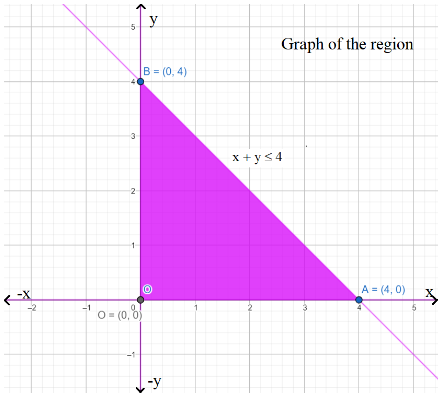Feasible Region having points O (0, 0), A (4, 0), and B (0, 4) at the corners

The points at the corners in the feasible region are O (0, 0), A (4, 0), and B (0, 4). Z assumes the following values on these points.

 Corner Point Z = 3x + 4y O(0,0) 0 A(4,0) 12 B(0,4) 16 → Maximum

Table of values

Thus, the maximum value of Z is 16 at the point B (0,4).

2. Minimize Z=-3x+4y subject to $\mathbf{x+2y\le 8}$, $\mathbf{3x+2y\le 12}$, $\mathbf{x\ge 0}$, $\mathbf{y\ge 0}$

Ans:  The given constraints are, $x+2y\le 8$, $3x+2y\le 12$, $x\ge 0$and $y\ge 0$, and the feasible region which is in accordance with the given constraints is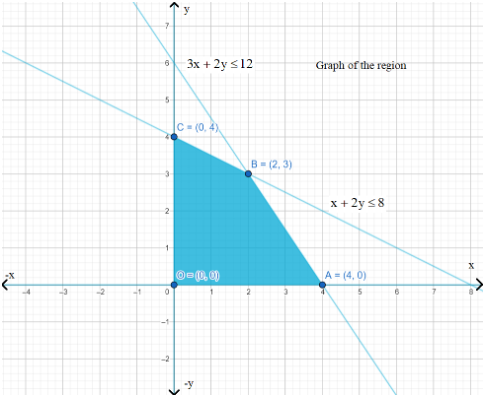Feasible Region having points O (0, 0), A (4, 0), B (2, 3), and C (0, 4) at the corners

The points at the corners in the feasible region are  O (0, 0), A (4, 0), B (2, 3), and C (0, 4). Z assumes the following values on these points.

 Corner Point Z=-3x+4y O(0,0) 0 A(4,0) -12 -> Minimum B(2,3) 6 C(4,0) 16

Thus, the minimum value of Z is -12 at the point (4, 0).

3.Maximize $\mathbf{Z=5x+3y}$ subject to $\mathbf{3x+5y\le 15}$, $\mathbf{5x+2y\le 10}$, $\mathbf{x\ge 0}$, $\mathbf{y\ge 0}$.

Ans: The given constraints are,$3x+5y\le 15$, $5x+2y\le 10$, $x\ge 0$, and $y\ge 0$, and the feasible region which is in accordance with the given constraints is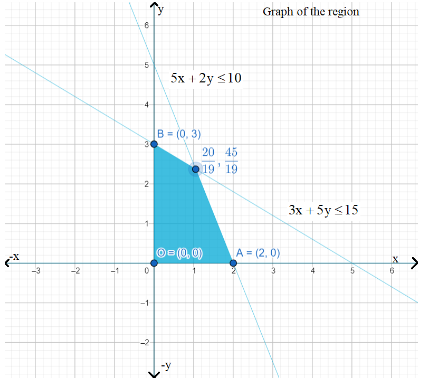Feasible Region having points O (0, 0), A (2, 0), B (0, 3), at the corners

The points at the corners in the feasible region are O (0, 0), A (2, 0), B (0, 3), and $C\left( \frac{20}{19},\frac{45}{19} \right)$. Z assumes the following values on these points.

 Corner Point Z=5x+3y O(0,0) 0 A(2,0) 10 B(0,3) 9 C(20/19,45/19) 235/18 -> Maximum

Thus, the maximum value of Z is $\frac{235}{19}$ at the point $\left( \frac{20}{19},\frac{45}{19} \right)$.

4.Minimize $Z=3x+5y$such that $\mathbf{x+3y\ge 3}$, $\mathbf{x+y\ge 2}$, $\mathbf{x,y\ge 0}$

Ans: The given constraints are, $x+3y\ge 3$, $x+y\ge 2$, and $x,y\ge 0$, and the feasible region which is in accordance with the given constraints is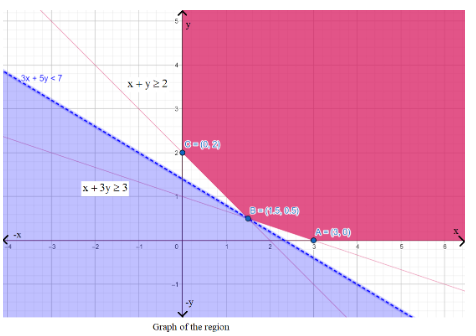Feasible Region having points A (0, 2), B (3, 0), at the corners

We can see that the feasible region is not bounded.

The points at the corners in the feasible region are A (3, 0), $B\left( \frac{3}{2},\frac{1}{2} \right)$ , and C (0, 2). Z assumes the following values on these points.

 Corner Point Z=3x+5y A(3,0) 9 B(3/2,½) 7 ->Smallest C(0,2) 10

Since the feasible region is unbounded, we cannot be sure that 7 is the minimum value of Z. To confirm this, we need to sketch the graph of the inequality, $3x+5y<7$, and see if the resulting plane has any point in common with the feasible region.

From the graph that we sketched, we can see that there is no common point between feasible regions and the sketched inequality $3x+5y<7$.

Z achieves minimum value 7  at $\left( \frac{3}{2},\frac{1}{2} \right)$.

5.Maximize Z=3x+2y subject to $\mathbf{x+2y\le 10}$, $\mathbf{3x+y\le 15}$, $\mathbf{x,y\ge 0}$.

Ans: The given constraints are,  $x+2y\le 10$, $3x+y\le 15$, $x\ge 0$, and $y\ge 0$, and the feasible region which is in accordance with the given constraints is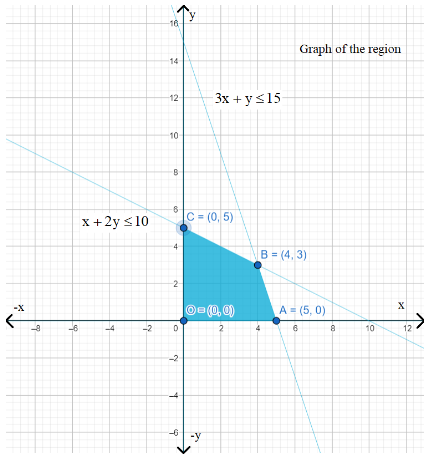Feasible Region having points A (5, 0), B (4, 3), and C (0, 5) at the corners

The points at the corners in the feasible region are A (5, 0), B (4, 3), and C (0, 5). Z assumes the following values on these points.

 Corner Point Z=3x+2y A(5,0) 15 B(4,3) 18 ->Maximum C(0,5) 10

Z achieves maximum value 18 at (4,3).

6.Minimize $Z=x+2y$subject to $2x+y\ge 3$, $x+2y\ge 6$, $x,y\ge 0$.

Ans:The given constraints are, $2x+y\ge 3$, $x+2y\ge 6$, $x\ge 0$, and $y\ge 0$, and the feasible region which is in accordance with the given constraints is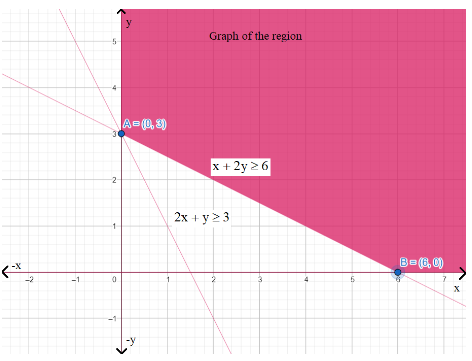Feasible Region having points A (6, 0) and B (0, 3) at the corners

The points at the corners in the feasible region are A (6, 0) and B (0, 3). Z assumes the following values on these points.

 Corner Point Z=x+2y A(6,0) 6 B(0,3) 6 ->Maximum

We can see that the value of Z is the same on both A and B hence, we will need to check on the other point on the line $x+2y=6$as well. The value of Z is 6 at point (2,2) also. Hence, the minimum value of Z Occurs at more than two points

Thus, the value of Z is minimum at every point on the line, $x+2y=6$

7. Minimize and Maximize Z=5x+10y subject to$\mathbf{x+2y\le 120}$, $\mathbf{x+y\ge 60}$, $\mathbf{x-2y\ge 0}$, $\mathbf{x,y\ge 0}$.

Ans:The given constraints are,  $x+2y\le 120$, $x+y\ge 60$, $x-2y\ge 0$, $x\ge 0$, and $y\ge 0$, and the feasible region which is in accordance with the given constraints is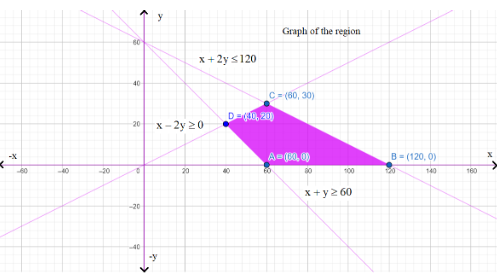Feasible Region having points A (60, 0), B (120, 0), C (60, 30), and D (40, 20) at the corners

The points at the corners in the feasible region are A (60, 0), B (120, 0), C (60, 30), and D (40, 20). Z assumes the following values on these points.

 Corner Point Z= 5x+10y A(60, 0) 300 →Minimum B(120, 0) 600 →Maximum C(60, 30) 600 →Maximum D(40,20) 400

Z achieves maximum and minimum values as 600 and 300 respectively. The point of maximum value is all the points on the line segment joining (120, 0) and (60, 30) and minimum value is (60,0).

8.Minimize and Maximize Z=x+2ysubject to $\mathbf{x+2y\ge 100}$, $\mathbf{2x-y\le 0}$, $\mathbf{2x+y\le 200}$, $\mathbf{x,y\ge 0}$.

Ans:The given constraints are, $x+2y\ge 100$, $2x-y\le 0$, $2x+y\le 200$, $x\ge 0$, and $y\ge 0$, and the feasible region which is in accordance with the given constraints is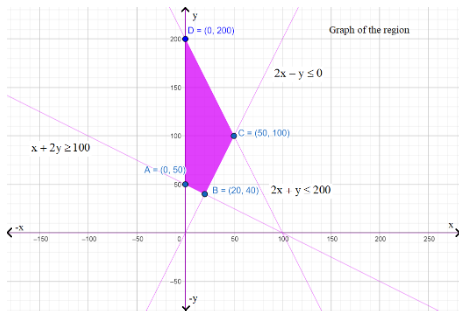Feasible Region having points A(0, 50), B(20, 40), C(50, 100), and D(0, 200) at the corners

The points at the corners in the feasible region are A(0, 50), B(20, 40), C(50, 100), and D(0, 200). Z assumes the following values on these points.

 Corner Point Z=x+2y A(0, 50) 100 →Minimum B(20,40) 100 →Minimum C(50,100) 250 D(0,200) 400 →Maximum

Z achieves maximum and minimum values as 400 and 100 respectively. The point of maximum value is (0,200) and minimum value is all points on the line joining the points (0, 50) and (20, 40).

9. Maximize Z=-x+2y, subject to the constraints: $\mathbf{x\ge 3}$, $\mathbf{x+y\ge 5}$, $\mathbf{x+2y\ge 6}$, $\mathbf{y\ge 0}$.

Ans: The given constraints are, $x\ge 3$, $x+y\ge 5$, $x+2y\ge 6$, and $y\ge 0$  and the feasible region which is in accordance with the given constraints is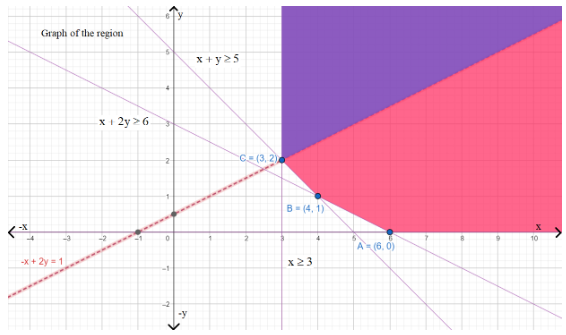Feasible Region having points A (6, 0), B (4, 1), and C (3, 2) at the corners

We can see that the feasible region is not bounded.

The points at the corners in the feasible region are A (6, 0), B (4, 1), and C (3, 2) . Z assumes the following values on these points.

 Corner Point Z=-x+2y A(6,0) Z=-6 B(4,1) Z=-2 C(3,2) Z=1

Since the feasible region is unbounded, we cannot be sure that 1 is the maximum value of Z. To confirm this, we need to sketch the graph of the inequality,  $-x+2y>1$, and see if the resulting plane has any point in common with the feasible region.

From the graph that we sketched, we can see that there are common points between feasible regions and the sketched inequality. Thus, Z = 1 is not the maximum value. Z has no maximum value.

10. Maximize Z=x+y , subject to$\mathbf{x-y\le -1}$, $\mathbf{-x+y\le 0}$, $\mathbf{x,y\ge 0}$.

Ans: The given constraints are, $x-y\le -1$, $-x+y\le 0$, $x,y\ge 0$and the feasible region which is in accordance with the given constraints is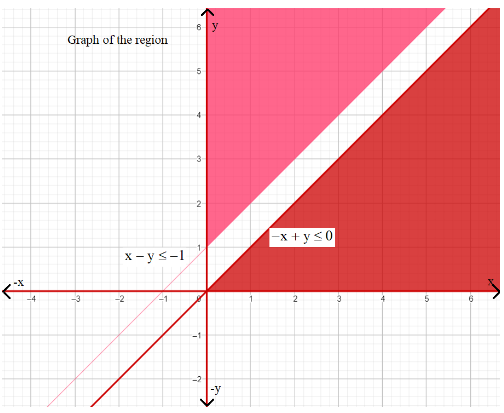Graph having No feasible region

We can see from the graph that there is no feasible region, hence Z has no maximum value.

### Exercise 12.2

1. Reshma wishes to mix two types of food P and Q in such a way that the vitamin contents of the mixture contain at least 8 units of vitamin A and 11 units of vitamin B. Food P costs Rs. 60/kg and Food Q costs Rs. 80/kg. Food P contains 3 units /kg of vitamin A and 5 units /kg of vitamin B while food Q contains 4 units /kg of vitamin A and 2 units /kg of vitamin B. Determine the minimum cost of the mixture?

Ans: Let the mixture contain $x$ kg of food P and $y$ kg of food Q. The constraints are, $x\ge 0$and $y\ge 0$

The given information is represented in the following table.

 Vitamin A(unit/kg) Vitamin B  (units/kg) Cost(Rs/kg) Food P 3 5 60 Food Q 4 2 80 Requirement(units/kg) 8 11

According to the question, the mixture must contain at least 8 units of vitamin A and 11 units of vitamin B.

Thus, the constraints are

$3x+4y\ge 8$

$5x+2y\ge 11$

Total cost, Z, of purchasing food can be represented as, $Z=60x+80y$

Mathematically this problem can be represented as

Minimize $Z=60x+80y$ … (1)

subject to the constraints,

$3x+4y\ge 8$… (2)

$5x+2y\ge 11$… (3)

$x,y\ge 0$… (4)

The graph of the feasible region in accordance with the constraints is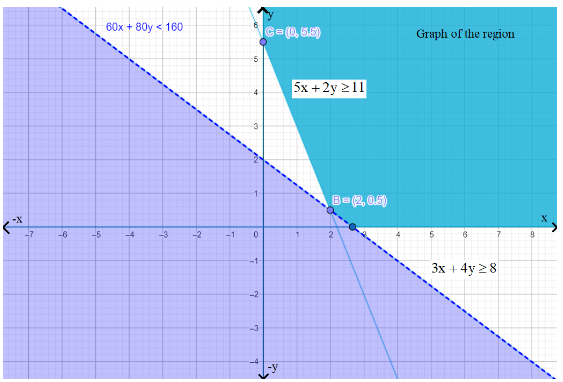Feasible Region having points B (2, 0.5), and C (0, 5.5) at the corners

We can see that the feasible region is not bounded. The points at the corners in the feasible region are$A\left( \frac{8}{3},0 \right)$, $B\left( 2,\frac{1}{2} \right)$and $C\left( 0,\frac{11}{2} \right)$.

Z assumes the following values on these points.

 Corner Point Z=60x +80y A($\frac{8}{3}$,0 ) 160 }→Minimum B(2, $\frac{1}{2}$ ) 160 C(0, $\frac{11}{2}$ ) 440

Since the feasible region is unbounded, we cannot be sure that 160  is the minimum value of Z. To confirm this, we need to sketch the graph of the inequality, $60x+80y<160$or $3x+4y<8$,  and see if the resulting plane has any point in common with the feasible region.

From the graph that we sketched, we can see that there is no common point between feasible regions and the sketched inequality $3x+4y<8$

Thus, the minimum cost of the mixture will be Rs. 160 at the line joining points $\left( \frac{8}{3},0 \right)$ and $\left( 2,\frac{1}{2} \right)$.

2. One kind of cake requires 200g flour and 25g of fat, and another kind of cake requires 100g of flour and 50g of fat. Find the maximum number of cakes which can be made from 5 kg of flour and 1 kg of fat assuming that there is no shortage of the other ingredients used in making the cakes?

Ans: Let us suppose there are $x$ cakes of first kind and $y$ cakes of the second kind. Therefore, $x\ge 0$and $y\ge 0$

The given information is represented in the following table.

 Flour(g) Fat(g) Cakes of first kind, x 200 25 Cakes of second kind, y 100 50 Availability 5000 1000

$\therefore 200x+100y\le 5000$

$\Rightarrow 2x+y\le 50$

$25x+50y\le 1000$

$\Rightarrow x+2y\le 40$

The total number of cakes can be represented by, $Z=x+y$

Mathematically we can represent the whole problem as

Maximize $Z=x+y$… (1)

subject to the constraints,

$2x+y\le 50$… (2)

$x+2y\le 40$… (3)

$x,y\ge 0$… (4)

The graph of the feasible region in accordance with the constraints is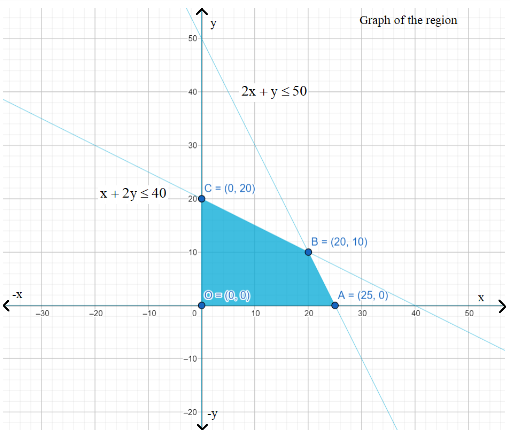Feasible Region having points A (25, 0), B (20, 10), O (0, 0), and C (0, 20) at the corners

We can see that the feasible region is not bounded. The points at the corners in the feasible region are A (25, 0), B (20, 10), O (0, 0), and C (0, 20). The values of Z at these corner points are as follows.

 Corner Point Z=x+y A(25, 0) 25 B(20, 10) 30 →Minimum C(0, 20) 20 O(0,0) 0

Thus, the maximum number of cakes that can be made are 30 (20 of one kind and 10 of the other kind).

3. A factory makes tennis rackets and cricket bats. A tennis racket takes 1.5 hours of machine time and 3 hours of craftsman’s time in its making while a cricket bat takes 3 hour of machine time and 1 hour of craftsman’s time. In a day, the factory has the availability of not more than 42 hours of machine time and 24 hours of craftsman’s time.

1. What number of rackets and bats must be made if the factory is to work at full capacity?

2. If the profit on a racket and on a bat is Rs. 20 and Rs. 10 respectively, find the maximum profit of the factory when it works at full capacity.

Ans:

1. Let the number of rackets to be made is  $x$ and the number of bats to be made is$y$ respectively. The available machine time is not more than 42 hours.

$\therefore 1.5x+3y\le 42$ … (1)

The craftsman’s time is not more than 24 hours.

$3x+y\le 24$… (2)

The factory is to work at full capacity. Therefore,

$1.5x+3y=42$

$3x+y=24$

Solving these equations, we obtain

$x=4$ and $y=12$

Thus, 4 rackets and 12 bats have to be made.

1. We can represent the given information in the following table.

 TennisRacket Cricket Bat Availability Machine Time(h) 1.5 3 42 Craftsman’s Time(h) 3 1 24

$\therefore 1.5x+3y\le 42$

$3x+y\le 24$

$x,y\ge 0$

The profit on racket is Rs. 20 and on  bat is Rs. 10.

Maximize $Z=20x+10y$...(1)

subject to the constraints,

$1.5x+3y\le 42$… (2)

$3x+y\le 24$… (3)

$x,y\ge 0$… (4)

The graph of the feasible region in accordance with the constraints is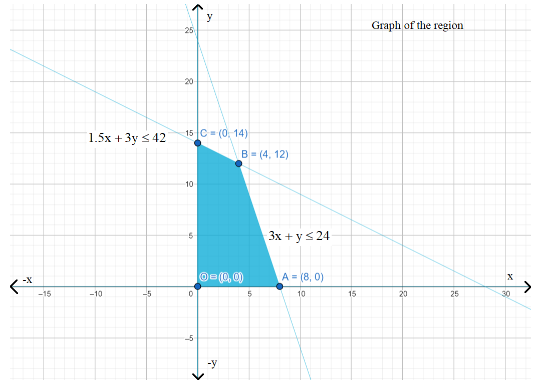Feasible Region having points A (8, 0), B (4, 12 ), C (0, 14), and O (0, 0) at the corners

The points at the corners in the feasible region are  A (8, 0), B (4, 12 ), C (0, 14), and O (0, 0). The values of Z at these corner points are as follows.

 Corner Point Z= 20x+10y A(8,0) 160 B(4,12) 200 →Minimum C(0,14) 140 O(0,0) 0

Thus, the maximum profit of the factory when it works to its full capacity is Rs. 200.

4. A manufacturer produces nuts and bolts. It takes 1 hour of work on machine A and 3 hours on machine B to produce a package of nuts. It takes 3 hours on machine A and 1 hour on machine B to produce a package of bolts. He earns a profit of Rs. 17.50 per package on nuts and Rs. 7.00 per package on bolts. How many packages of each should be produced each day so as to maximize his profit, if he operates his machines for at the most 12 hours a day?

Ans:  Let there be $x$ packages of nuts and $y$ packages of bolts. The constraints are, $x\ge 0$and $y\ge 0$

We can represent the following information in the form of a table.

 Nuts Bolts Availability Machine A(h) 1 3 12 Machine B(h) 3 1 12

The profit on a package of nuts is Rs. 17.50 and on a package of bolts is Rs. 7. Therefore, the constraints are

$x+3y\le 12$

$3x+y\le 12$

Total profit, $Z=17.5x+7y$

Mathematically we can formulate this problem as

Maximize $Z=17.5x+7y$… (1)

subject to the constraints,

$x+3y\le 12$… (2)

$3x+y\le 12$… (3)

$x,y\ge 0$… (4)

The graph of the feasible region in accordance with the constraints is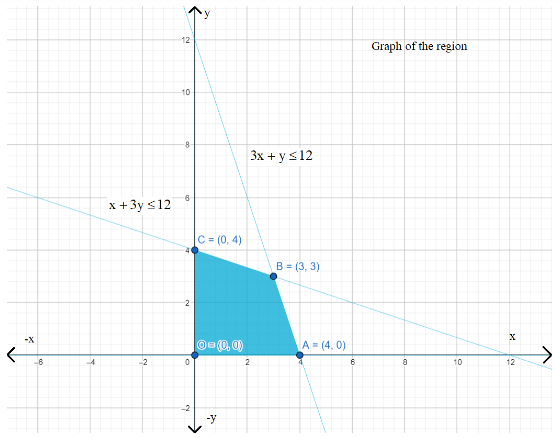Feasible Region having points   A (4, 0), B (3,3), C (0, 4), and O (0, 0) at the corners

The points at the corners in the feasible region are  A (4, 0), B (3,3), C (0, 4), and O (0, 0). The values of Z at these corner points are as follows.

 Corner Point Z=17.5 x + 7y A(4,0) 70 B(3,3) 73.5 →Minimum C(0,4) 28 D(0,0) 0

The maximum value of Z is Rs. 73.50 at point (3, 3).

5. A factory manufactures two types of screws, A and B. Each type of screw requires the use of two machines, an automatic and a hand operated. It takes 4 minutes on the automatic and 6 minutes on hand operated machines to manufacture a package of screws A, while it takes 6 minutes on automatic and 3 minutes on the hand operated machines to manufacture a package of screws B. Each machine is available for at most 4 hours on any day. The manufacturer can sell a package of screws A at a profit of Rs. 7 and screws B at a profit of Rs10. Assuming that he can sell all the screws he manufactures, how many packages of each type should the factory owner produce in a day in order to maximize his profit? Determine the maximum profit.

Ans: Let there be $x$ screws of type A and $y$ screws of type B manufactured on each day. Therefore,

$x\ge 0$and $y\ge 0$

We can represent the given information in the form of a table as follows.

 Screw A Screw B Availability Automatic Machine(min) 4 6 4 $\times$ 60 = 240 Hand Operated Machine(min) 6 3 4 $\times$ 60 = 240

The profit on a package of screws A is Rs. 7 and on the package of screws B is Rs. 10.

Therefore, the constraints are

$4x+6y\le 240$

$6x+3y\le 240$

Total profit, $Z=7x+10y$

Mathematically this problem can be formulated as follows

Maximize $Z=7x+10y$… (1)

subject to the constraints

$4x+6y\le 240$… (2)

$6x+3y\le 240$… (3)

$x,y\ge 0$… (4)

The graph of the feasible region in accordance with the constraints is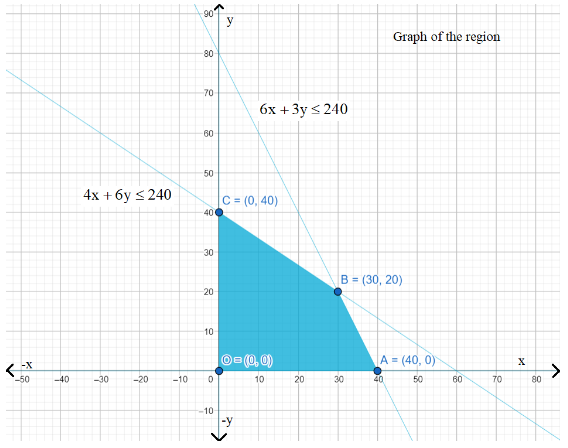Feasible Region having points A (40, 0), B (30, 20), and C (0, 40) at the corners

The points at the corners in the feasible region are  A (40, 0), B (30, 20), and C (0, 40). The values of Z at these corner points are as follows.

 Corner Point Z= 7x  + 10y A(40,0) 280 B(30, 20) 410 →Minimum C(0,40) 400

The maximum value of Z is 410 at point (30, 20)

6. A cottage industry manufactures pedestal lamps and wooden shades, each requiring the use of a grinding /cutting machine and a sprayer. It takes 2 hours on a grinding /cutting machine and 3 hours on the sprayer to manufacture a pedestal lamp. It takes 1 hour on the grinding /cutting machine and 2 hours on the sprayer to manufacture a shade. On any day, the sprayer is available for at the most 20 hours and the grinding/cutting machine for at the most 12 hours. The profit from the sale of a lamp is Rs. 5 and that from a shade is Rs. 3. Assuming that the manufacturer can sell all the lamps and shades that he produces, how should he schedule his daily production in order to maximize his profit?

Ans: Let there be $x$ pedestal lamps and $y$ wooden shades manufactured. The constraints are, $x\ge 0$ and $y\ge 0$

We can represent the given information as the following table.

 Lamps Shades Availability Grinding/Cutting Machine(h) 2 1 12 Sprayer(h) 3 2 20

The profit on a lamp is Rs. 5 and on the shades is Rs. 3.

Therefore, the constraints are

$2x+y\le 12$

$3x+2y\le 20$

Total profit, $Z=5x+3y$

Mathematically we can formulate the problem as

Maximize $Z=5x+3y$… (1)

subject to the constraints,

$2x+y\le 12$ … (2)

$3x+2y\le 20$ … (3)

$x,y\ge 0$ … (4)

The graph of the feasible region in accordance with the constraints is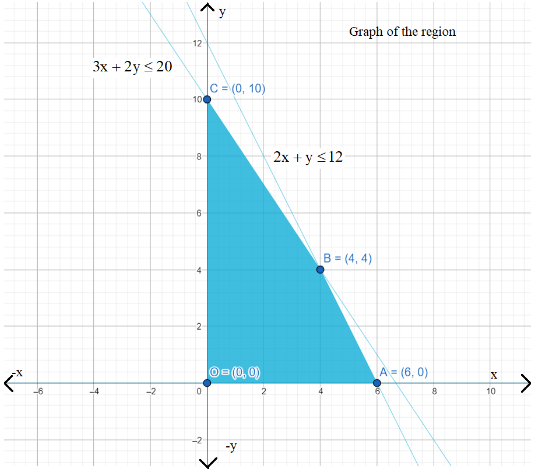Feasible Region having points A (6, 0), B (4, 4), and C (0, 10) at the corners

The points at the corners in the feasible region are A (6, 0), B (4, 4), and C (0, 10). The values of Z at these corner points are as follows.

 Corner Point Z = 5x + 3y A(6,0) 30 B(4,4) 32 →Minimum C(0, 10) 30

The maximum value of Z is 32 at point (4, 4).

7. A company manufactures two types of novelty Souveniers made of plywood. Souveniers of type A require 5 minutes each for cutting and 10 minutes each for assembling. Souveniers of type B require 8 minutes each for cutting and 8 minutes each for assembling. There are 3 hours 20 minutes available for cutting and 4 hours of assembling. The profit is Rs. 5 each for type A and Rs. 6 each for type B Souveniers. How many Souveniers of each type should the company manufacture in order to maximize the profit?

Ans: Let there be $x$ Souveniers of type A and $y$ Souveniers of type B. The constraints are, $x\ge 0$and $y\ge 0$

We can represent the given information in the table as follows

 Type A Type B Availability Cutting(min) 5 8 3 $3 \times 60 +20=200$ Assembling (min) 10 8 $4 \times 60 = 240$

The profit on type A Souveniers is Rs. 5 and on type B Souveniers is Rs. 6. Therefore, the constraints are

$5x+8y\le 200$

$10x+8y\le 240$i.e., $5x+4y\le 120$

Total profit, $Z=5x+6y$

Mathematically we can formulate the whole problem as

Maximize $Z=5x+6y$… (1)

subject to the constraints,

$5x+8y\le 200$… (2)

$5x+4y\le 120$… (3)

$x,y\ge 0$ ... (4)

The graph of the feasible region in accordance with the constraints is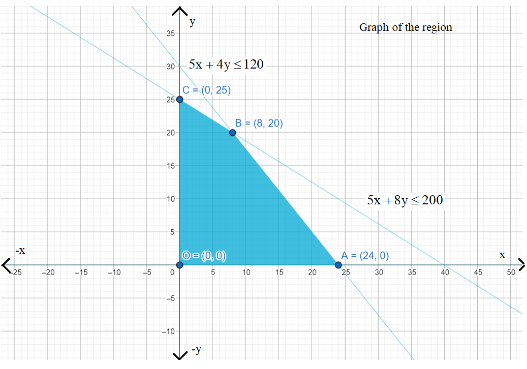Feasible Region having points  A (24, 0), B (8, 20), and C (0, 25) at the corners

The points at the corners in the feasible region are A (24, 0), B (8, 20), and C (0, 25). The values of Z at these corner points are as follows.

 Corner Point Z = 5x + 6y A(24, 0) 120 B(8, 20) 160 →Minimum C(0,25) 150

The maximum value of Z is 200 at point (8, 20).

8. A merchant plans to sell two types of personal computers − a desktop model and a portable model that will cost Rs. 25000 and Rs. 40000 respectively. He estimates that the total monthly demand of computers will not exceed 250 units. Determine the number of units of each type of computer which the merchant should stock to get maximum profit if he does not want to invest more than Rs. 70 lakhs and if his profit on the desktop model is Rs. 4500 and on the portable model is Rs. 5000.

Ans: Let there be $x$ desktop models and $y$ portable models stocked by merchants. Therefore, $x\ge 0$and $y\ge 0$

The cost of a desktop model is Rs. 25000 and of a portable model is Rs. 4000. The merchant can only invest a maximum of Rs. 70 lakhs.

$\therefore 25000x+40000y\le 7000000$

$5x+8y\le 1400$

The monthly demand of computers will not exceed 250 units.

$\therefore x+y\le 250$

The profit on a desktop model is Rs. 4500 and the profit on a portable model is Rs. 5000.

Total profit, $Z=4500+5000y$

Mathematically this problem can be formulated as

Maximum $Z=4500+5000y$ … (1)

Subject to the constraints,

$5x+8y\le 1400$… (2)

$x+y\le 250$ … (3)

$x,y\ge 0$ … (4)

The graph of the feasible region in accordance with the constraints is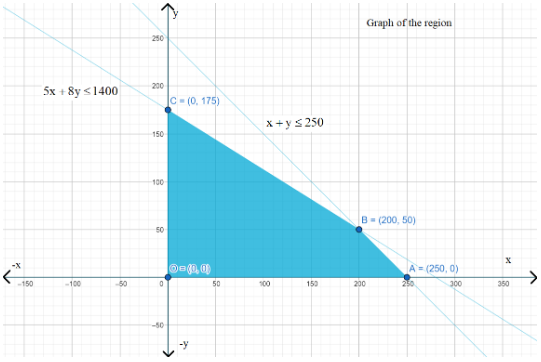Feasible Region having points A (250, 0), B (200, 50), and C (0, 175) at the corners

The points at the corners in the feasible region are A (250, 0), B (200, 50), and C (0, 175).

The values of Z at these corner points are as follows.

 Corner Point Z = 4500 + 5000y A(250, 0) 1125000 B(200, 50) 1150000 →Minimum C(0. 175) 875000

The maximum value of Z is 1150000 at point (200, 50).

9. A diet is to contain at least 80 units of vitamin A and 100 units of minerals. Two foods ${{F}_{1}}$ and ${{F}_{2}}$ are available. Food ${{F}_{1}}$ costs Rs. 4 per unit food and ${{F}_{2}}$ costs Rs. 6 per unit. One unit of food ${{F}_{1}}$ contains 3 units of vitamin A and 4 units of minerals. One unit of food ${{F}_{2}}$ contains 6 units of vitamin A and 3 units of minerals. Formulate this as a linear programming problem. Find the minimum cost for a diet that consists of a mixture of these two foods and also meets the minimal nutritional requirements?

Ans: Let there be $x$ units of food $F_1$ and y units of food $F_2$ in the diet. Therefore, $x\ge 0$and $y\ge 0$

We can represent the given information in the table as follows

 Vitamin A Mineral (units) Cost Per Unit(Rs) Food $F_1$(X) 3 4 4 Food F_2 (y) 6 3 6 Requirement 80 100

The cost of food ${{F}_{1}}$, is Rs. 4 per unit and of Food ${{F}_{2}}$ is Rs. 6 per unit.

Therefore, the constraints are

$3x+6y\ge 80$

$4x+3y\ge 100$

$x,y\ge 0$

Total cost of the diet, $Z=4x+6y$

Mathematically this problem can be formulated as

Minimize $Z=4x+6y$… (1)

subject to the constraints,

$3x+6y\ge 80$… (2)

$4x+3y\ge 100$… (3)

$x,y\ge 0$… (4)

The graph of the feasible region in accordance with the constraints is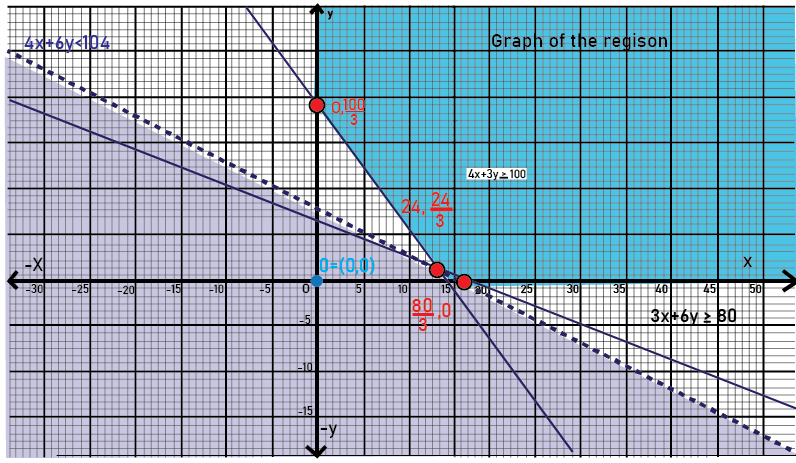Feasible Region having points  (24,24) at the corners

We can see that the feasible region is not bounded.

The points at the corners in the feasible region are$A\left( \frac{80}{3},0 \right)$, $B\left( 24,\frac{4}{3} \right)$, and $C\left( 0,\frac{100}{3} \right)$.

The values of Z at these corner points are as follows.

 Concern Point Z = 4x + 6y A($\frac{80}{3}$, 0) $\frac{320}{3} = 106.67$ B(24, $\frac{4}{3}$) 104 →Minimum C= (0, $\frac{100}{3}$) 200

Since the feasible region is unbounded, we cannot be sure that 104 is the maximum value of Z. To confirm this, we need to sketch the graph of the inequality, $4x+6y<104$or $2x+3y<52$, and see if the resulting plane has any point in common with the feasible region.

From the graph that we sketched, we can see that there are no common points between feasible regions and the sketched inequality 2x+3y < 52

Therefore, the minimum cost of the mixture will be Rs. 104.

10. There are two types of fertilizers ${{F}_{1}}$ and ${{F}_{2}}$. ${{F}_{1}}$ consists of 10% nitrogen and 6% phosphoric acid and ${{F}_{2}}$ consists of 5% nitrogen and 10% phosphoric acid. After testing the soil conditions, a farmer finds that she needs at least 14 kg of nitrogen and 14 kg of phosphoric acid for her crop. If ${{F}_{1}}$ cost Rs. 6/kg and ${{F}_{2}}$ costs Rs. 5/kg, determine how much of each type of fertilizer should be used so that nutrient requirements are met at a minimum cost. What is the minimum cost?

Ans: Let there be $x$ kg of fertilizer ${{F}_{1}}$and $y$ kg of fertilizer ${{F}_{2}}$bought by the farmer. Therefore, $x\ge 0$and $y\ge 0$

We can represent the given information in the form of a table as follows.

 Nitrogen(%) Phosphoric Acid (%) Cost (Rs/ kg) $F_1(x)$ 10 6 6 $F_2( y)$ 5 10 5 Requirement (kg) 14 14

${{F}_{1}}$consists of 10% nitrogen and ${{F}_{2}}$consists of 5% nitrogen. The farmer requires at least 14 kg of nitrogen.

$\therefore 10%$of $x+5%$ of $y\ge 14$

$\frac{x}{10}+\frac{y}{20}\ge 14$

$2x+y\ge 280$

${{F}_{1}}$consists of $6%$ phosphoric acid and ${{F}_{2}}$consists of $10%$ phosphoric acid. The farmer requires at least 14 kg of phosphoric acid.

$\therefore 6%$ of $x+10%$ of $y\ge 14$

$\frac{6x}{100}+\frac{10y}{100}\ge 14$

$3x+56y\ge 700$

Total cost of fertilizers, $Z=6x+5y$

Mathematically, we can formulate this problem as

Minimize $Z=6x+5y$… (1)

subject to the constraints,

$2x+y\ge 280$… (2)

$3x+5y\ge 700$… (3)

$x,y\ge 0$… (4)

The graph of the feasible region in accordance with the constraints is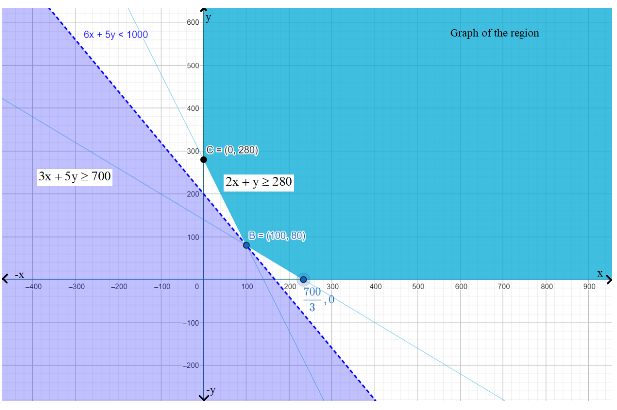Feasible Region having points  B(100,80) , C(0,280) at the corners

We can see that the feasible region is not bounded.

The points at the corners in the feasible region are$A\left( \frac{700}{3},0 \right)$, $B\left( 100,80 \right)$, and $C\left( 0,280 \right)$

The values of Z at these corner points are as follows.

 Corner Point Z = 6x + 5y A($\frac{700}{3}$, 0) 1400 B(100, 80 ) 1000 $\rightarrow Minimum$ C(0 , 280) 1400

Since the feasible region is unbounded, we cannot be sure that 1000 is the minimum value of Z. To confirm this, we need to sketch the graph of the inequality,$6x+5y<1000$, and see if the resulting plane has any point in common with the feasible region. From the graph that we sketched, we can see that there are no common points between feasible regions and the sketched inequality $6x+5y<1000$

Thus, 100 kg of fertilizer ${{F}_{1}}$and 80 kg of fertilizer ${{F}_{2}}$should be used to minimize the cost. The minimum cost is Rs. 1000.

11. The corner points of the feasible region determined by the following system of linear inequalities:

$2x+y\le 10$, $x+3y\le 15$, $xy\ge 0$ are $\left( 0,0 \right)$, $\left( 5,0 \right)$, $\left( 3,4 \right)$, and $\left( 0,5 \right)$. Let $Z=px+qy$where $p,q>0$. Condition on p and q so that the maximum of Z occurs at both $\left( 3,4 \right)$and $\left( 0,5 \right)$ is

1. $p=q$

2. $p=2q$

3. $p=3q$

4. $q=3p$

Ans: The maximum value of Z should be unique.

We are  given that the maximum value of Z occurs at two points, $\left( 3,4 \right)$and $\left( 0,5 \right)$.

$\therefore$ Value of Z at $\left( 3,4 \right)$= Value of Z at $\left( 0,5 \right)$

$\Rightarrow p(3)+q(4)=p(0)+q(5)$

$\Rightarrow 3p+4q=5q$

$\Rightarrow q=3p$

### Miscellaneous - Exercise

1. Refer to Example 9. How many packets of each food should be used to maximize the amount of vitamin A in the diet? What is the maximum amount of vitamin A in the diet?

Ans: Let there be $x$ and $y$ packets of foods P and Q respectively in the diet.

Thus,

$x\ge 0$and $y\ge 0$

Mathematically this problem can be formulated as

Maximize $z=6x+3y$… (1)

subject to the constraints,

$4x+y\ge 80$ … (2)

$x+5y\ge 115$ … (3)

$3x+2y\le 150$ … (4)

$x,y\ge 0$ … (5)

The graph of the feasible region in accordance with the constraints is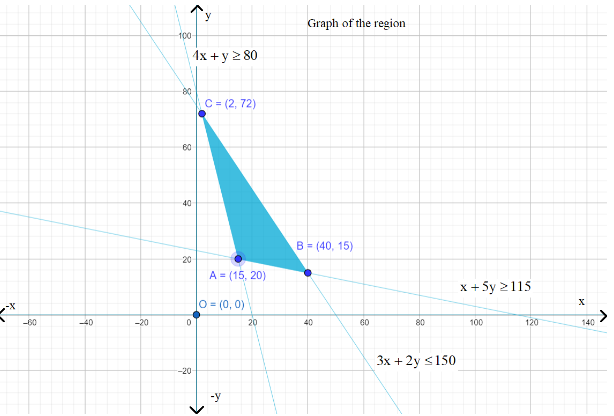Feasible Region having points A(15,20),  B(40,15) , C(2,72) at the corners

The points at the corners in the feasible region are A (15, 20), B (40, 15), and C (2, 72).

The values of Z at these corner points are as follows.

 Corner Point z = 6x + 3y A(15, 20) 150 B(40, 15) 285 $\rightarrow Maximum$ C(2, 72) 228

Thus, the maximum value of z is 285 at point (40, 15).

2. A farmer mixes two brands P and Q of cattle feed. Brand P, costing Rs. 250 per bag contains 3 units of nutritional element A, 2.5 units of element B and 2 units of element C. Brand Q costing Rs. 200 per bag contains 1.5 units of nutritional elements A, 11.25 units of element B, and 3 units of element C. The minimum requirements of nutrients A, B and C are 18 units, 45 units and 24 units respectively. Determine the number of bags of each brand which should be mixed in order to produce a mixture having a minimum cost per bag? What is the minimum cost of the mixture per bag?

Ans: Let there be $x$ bags of brand P and $y$ bags of brand Q mixed by the farmer. We can represent the given information in the form of a table as follows.

 Vitamin A (units/kg) Vitamin B (units/kg) Cost(Rs/kg) Food P 3 5 60 Food Q 4 2 80 Requirements(units/kg) 8 11

We can formulate the problem as follows

Minimize $z=250x+200y$… (1)

subject to the constraints,

$3x+1.5y\ge 18$ … (2)

$2.5x+11.25y\ge 45$ … (3)

$2x+3y\ge 24$ … (4)

$x,y\ge 0$ … (5)

The graph of the feasible region in accordance with the constraints is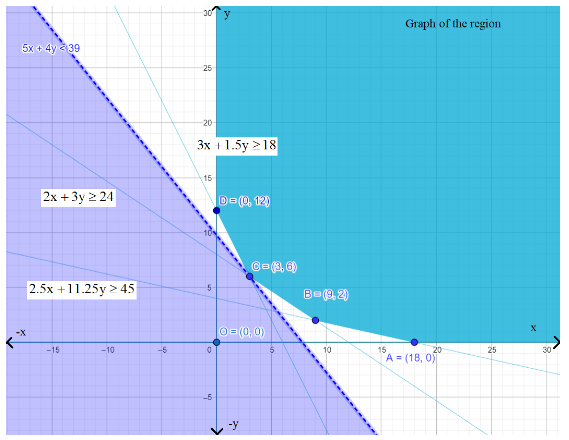Feasible Region having points A(18,0),  B(9,2) , C(3,6) and D(0,12) at the corners

The points at the corners in the feasible region are A (18, 0), B (9, 2), C (3, 6), and D (0, 12). The values of Z at these corner points are as follows.

 Corner Point Z = 250x +200 y A(18, 0) 4500 B(9, 2) 2650 C(3, 6) 1950 $\rightarrow Minimum$ D(0, 12) 2400

Since the feasible region is unbounded, we cannot be sure that 1950 is the minimum value of Z. To confirm this, we need to sketch the graph of the inequality, $250x+200y<1950$or $5x+4y<39$,  and see if the resulting plane has any point in common with the feasible region.

From the graph that we sketched, we can see that there are no common points between feasible regions and the sketched inequality $5x+4y<39$

Thus, the minimum value of z is 2000 at point (3, 6).

3. A dietician wishes to mix together two kinds of food X and Y in such a way that the mixture contains at least 10 units of vitamin A, 12 units of vitamin B and 8 units of vitamin C. The vitamin content of one kg food is given below:

 Food Vitamin A Vitamin B Vitamin C X 1 2 3 Y 2 2 1

One kg of food X costs Rs. 16 and one kg of food Y costs Rs. 20. Find the least cost of the mixture which will produce the required diet?

Ans: Let there be $x$ kg of food X and $y$ kg of food Y in the mixture.

Mathematically this problem can be formulated as follows.

Minimize $z=16x+20y$… (1)

subject to the constraints,

$x+2y\ge 10$ … (2)

$x+y\ge 6$ … (3)

$3x+y\ge 8$ … (4)

$x,y\ge 0$ … (5)

The graph of the feasible region in accordance with the constraints is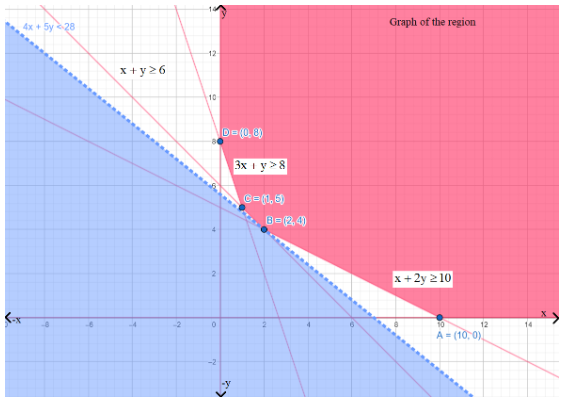Feasible Region having points A(10,0),  B(2,4) , C(1,5) and D(0,8) at the corners

The points at the corners in the feasible region are A (10, 0), B (2, 4), C (1, 5), and D (0, 8).

The values of Z at these corner points are as follows.

 Corner Point Z = 16x + 20y A(10, 0) 160 B(2, 4) 112 C(1, 5) 116 $\rightarrow Minimum$ D(0, 8) 160

Since the feasible region is unbounded, we cannot be sure that 112is the minimum value of Z. To confirm this, we need to sketch the graph of the inequality, $16x+20y<112$ or $4x+5y<28$,and see if the resulting plane has any point in common with the feasible region.

From the graph that we sketched, we can see that there are no common points between feasible regions and the sketched inequality  $4x+5y<28$

Thus, the minimum value of Z is 112 at point (2, 4).

4.A manufacturer makes two types of toys A and B. Three machines are needed for this purpose and the time (in minutes) required for each toy on the machines is given below:

 Type of Toys Machines $\textrm{I}$ $\textrm{II}$ $\textrm{III}$ A 12 18 6 B 6 0 9

Each machine is available for a maximum of 6 hours per day. If the profit on each toy of type A is Rs. 7.50 and that on each toy of type B is Rs. 5, show that 15 toys of type A and 30 of type B should be manufactured in a day to get maximum profit.

Ans: Let $x$ and $y$ toys of type A and type B respectively are manufactured in a day. Mathematically this problem can be formulated as follows

Maximize $z=7.5x+5y$… (1)

subject to the constraints,

$2x+y\le 60$

$x\le 20$

$2x+3y\le 120$

$x,y\ge 0$

The graph of the feasible region in accordance with the constraints is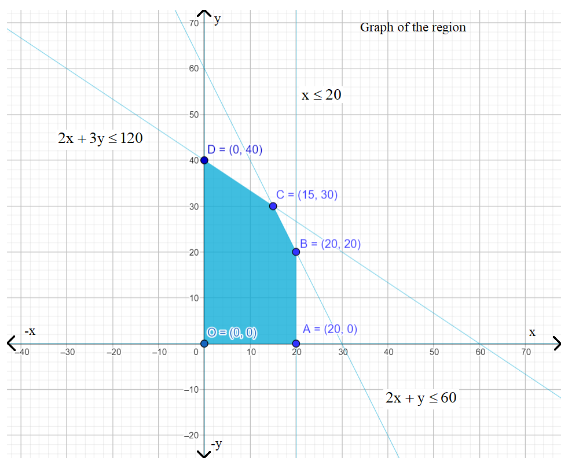Feasible Region having points  A (20, 0), B (20, 20), C (15, 30), and D (0, 40) at the corners

The points at the corners in the feasible region are A (20, 0), B (20, 20), C (15, 30), and D (0, 40). The values of Z at these corner points are as follows.

 Corner Point Z= 7.5 x +5y A(20, 0) 150 B(20, 20) 250 C(15, 30) 262.5 $\rightarrow maximum$ D(0, 40) 200

Thus, The maximum value of z is 262.5 at point (15, 30).

5. An aeroplane can carry a maximum of 200 passengers. A profit of Rs. 1000 is made on each executive class ticket and a profit of Rs. 600 is made on each economy class ticket. The airline reserves at least 20 seats for executive class. However, at least 4 times as many passengers prefer to travel by economy class than by the executive class. Determine how many tickets of each type must be sold in order to maximize the profit for the airline. What is the maximum profit?

Ans: Let there be $x$ tickets of executive class and $y$ tickets for economy class sold by the airline. Mathematically this problem can be formulated as follows

Maximize $z=1000x+600y$… (1)

subject to the constraints,

$x+y\le 200$ … (2)

$x\ge 20$ … (3)

$y-4x\ge 0$ … (4)

$x,y\ge 0$ … (5)

The graph of the feasible region in accordance with the constraints is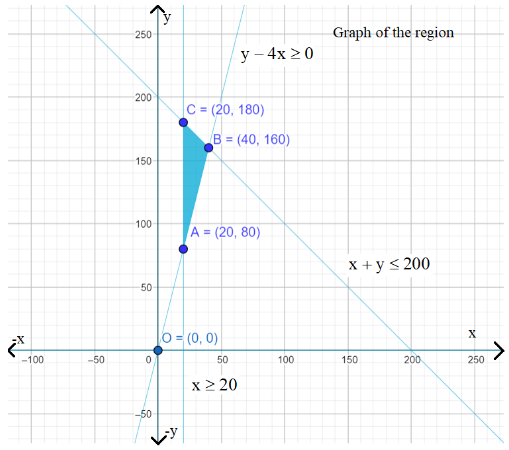Feasible Region having points  A (20, 80), B (40, 160), and C (20, 180) at the corners

The points at the corners in the feasible region are A (20, 80), B (40, 160), and C (20, 180). The values of Z at these corner points are as follows.

 Corner Point Z = 1000x + 600 y A(20, 80) 68000 B(40, 160) 136000 $\rightarrow maximum$ C(20, 180) 128000

Thus, the maximum value of z is 136000 at point (40, 160).

6. Two godowns A and B have grain capacity of 100 quintals and 50 quintals respectively. They supply to 3 ration shops, D, E and F whose requirements are 60, 50 and 40 quintals respectively. The cost of transportation per quintal from the godowns to the shops are given in the following table:

 Transportation Cost Per Quintal(in Rs) From/To A B DEF 632.50 432

How should the supplies be transported in order that the transportation cost is minimum? What is the minimum cost?

Ans: Let godown A supply $x$ and $y$ quintals of grain to the shops D and E respectively. Then, $\left( 100-x-y \right)$ quintals of grain are to be supplied to shop F.

The requirement at shop D is 60 quintals . Since $x$ quintals are transported from godown A, the remaining $\left( 60-x \right)$ quintals will be transported from godown B. Similarly, $\left( 50-y \right)$ quintals and $40-\left( 100-x-y \right)=\left( x+y-60 \right)$quintals will be transported from godown B to shop E and F respectively.

We can represent the given information in the form of a diagram as follows.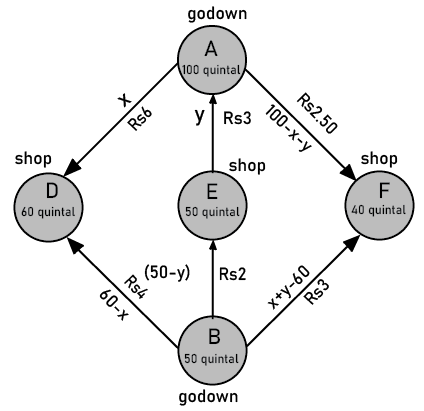Godown A ,B,C,D,E,F shown

$x\ge 0$, $y\ge 0$, and $100-x-y\ge 0$

$\Rightarrow x\ge 0$, $y\ge 0$, and $x+y\le 100$

$60-x\ge 0$, $50-y\ge 0$and $x+y-60\ge 0$

$\Rightarrow x\le 60$, $y\le 50$, and $x+y\ge 60$

We can calculate the total transportation cost Z as follows

$z=6x+3y+2.5\left( 100-x-y \right)+4\left( 60-x \right)+2\left( 50-y \right)+3\left( x+y-60 \right)$

$=6x+3y+250-2.5x-2.5y+240-4x+100-2y+3x+3y-180$

$=2.5x+1.5y+410$

We can formulate the given problem as

Minimize $z=2.5x+1.5y+410$… (1)

subject to the constraints,

$x+y\le 100$

$x\le 60$

$y\le 50$

$x+y\ge 60$

$x,y\ge 0$

The graph of the feasible region in accordance with the constraints isFeasible Region having points   A (60, 0), B (60, 40), C (50, 50), and D (10, 50) at the corners

The points at the corners in the feasible region are A (60, 0), B (60, 40), C (50, 50), and D (10, 50). The values of Z at these corner points are as follows.

 Corner Point Z = 2.5 x + 1.5 y + 410 A(60, 0) 560 B(60, 40) 620 C(50, 50) 610 D(10, 50) 510 $\rightarrow minimum$

Thus, the minimum value of z is 510 at point (10, 50).

7. An oil company has two depots A and B with capacities of 7000 L and 4000 L respectively. The company is to supply oil to three petrol pumps, D, E and F whose requirements are 4500L, 3000L and 3500L respectively. The distance (in km) between the depots and the petrol pumps is given in the following table: Assuming that the transportation cost of 10 litres of oil is Rs. 1 per km, how should the delivery be scheduled in order that the transportation cost is minimum? What is the minimum cost?

Ans: Let $x$ and $y$ litres of oil be supplied from A to the petrol pumps, D and E. Then, $\left( 7000-x-y \right)$will have to be supplied from A to petrol pump F.

The requirement at petrol pump D is 4500 L. Since, $x$ L are transported from depot A, the remaining $\left( 4500-x \right)$ L will be transported from petrol pump B.

Similarly, $\left( 3000-y \right)$L and $3500-\left( 7000-x-y \right)=\left( x+y-3500 \right)$ L will be transported from depot B to petrol pump E and F respectively.

We can represent the given information in the form of a diagram as follows.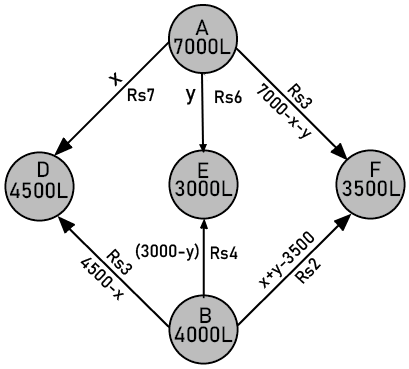Depots A and B with 7000L and 4000L

$x\ge 0$, $y\ge 0$, and $\left( 7000-x-y \right)\ge 0$

$\Rightarrow x\ge 0$, $y\ge 0$, and $x+y\le 7000$

$4500-x\ge 0$, $3000-y\ge 0$, and $x+y-3500\ge 0$

$\Rightarrow x\le 4500$, $y\le 3000$, and $x+y\ge 3500$

Cost of transporting 10 L of petrol = Rs. 1

Cost of transporting 1 L of petrol = $\frac{1}{10}$

Therefore, we can calculate the total transportation cost as follows,

$z=\frac{7}{10}\times x+\frac{6}{10}y+\frac{3}{10}\left( 7000-x-y \right)+\frac{3}{10}\left( 4500-x \right)+\frac{4}{10}\left( 3000-y \right)+\frac{2}{10}\left( x+y-3500 \right)$

$=0.3x+0.1y+3950$

We can formulate this problem as follows

Minimize $z=0.3x+0.1y+3950$… (1)

subject to the constraints,

$x+y\le 7000$ … (2)

$x\le 4500$ … (3)

$y\le 3000$ … (4)

$x+y\ge 3500$ … (5)

$x,y\ge 0$ … (6)

The feasible region determined by the constraints is given by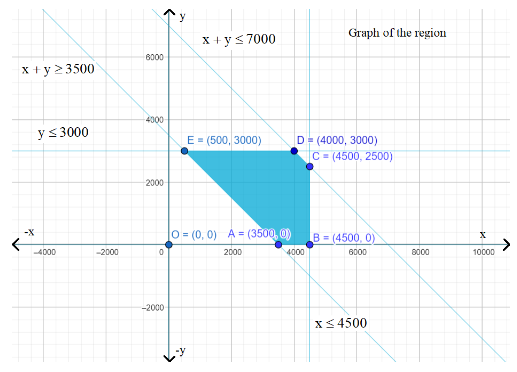Feasible Region having points  A (3500, 0), B (4500, 0), C (4500, 2500), D (4000, 3000), and E (500, 3000) at the corners

The points at the corners in the feasible region areA (3500, 0), B (4500, 0), C (4500, 2500), D (4000, 3000), and E (500, 3000). The values of Z at these corner points are as follows.

 Corner Point Z = 0.3 x + 0.1 y +3950 A(3500, 0) 5000 B(4500, 0) 5300 C(4500, 2500) 5550 D(4000, 3000) 5450 E(500, 3000) 4400

Thus, the minimum value of z is 4400 at point (500, 3000).

8. A fruit grower can use two types of fertilizer in his garden, brand P and brand Q. The amounts (in kg) of nitrogen, phosphoric acid, potash, and chlorine in a bag of each brand are given in the table. Tests indicate that the garden needs at least 240 kg of phosphoric acid, at least 270 kg of potash and at most 310 kg of chlorine. If the grower wants to minimize the amount of nitrogen added to the garden, how many bags of each brand should be used? What is the minimum amount of nitrogen added in the garden?

 KG Per Bag Brand P Brand Q Nitrogen 3 3.5 Phosphoric acid 1 2 Potash 3 1.5 chlorine 1.5 2

Ans:  Let there be $x$ bags of brand P and $y$ bags of brand Q used by fruit grower. We can formulate this problem as follows

Minimize $z=3x+3.5y$… (1)

Subject to constraints,

$x+2y\ge 240$ … (2)

$x+0.5y\ge 90$ … (3)

$1.5x+2y\le 310$ … (4)

$x,y\ge 0$ … (5)

The feasible region determined by the constraints is given by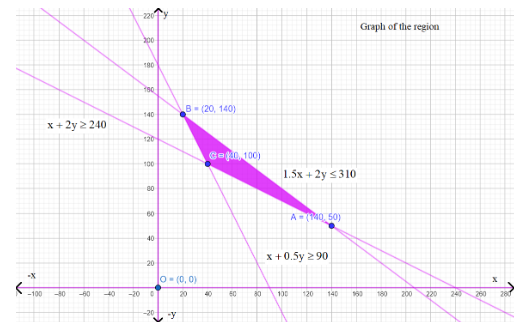Feasible Region having points A (240, 0), B (140, 50), and C (20, 140) at the corners

The points at the corners in the feasible region are A (240, 0), B (140, 50), and C (20, 140). The values of Z at these corner points are as follows.

 Corner Point Z = 3x+3.5y A(140, 50) 595 B(20, 140) 550 C(40, 100) 470 $\rightarrow minimum$

Thus, the maximum value of z is 470 at point (40, 100).

9. Refer to question 8. If the grower wants to maximize the amount of nitrogen added to the garden, how many bags of each brand should be added? What is the maximum amount of nitrogen added?

Ans: Let there be $x$ bags of brand P and $y$ bags of brand Q used by the farm grower.

We can formulate this problem as

Maximize $z=3x+3.5y$… (1)

subject to the constraints,

$x+2y\ge 240$

$x+0.5y\ge 90$

$1.5x+2y\le 310$

$x,y\ge 0$

The feasible region determined by the constraints is given byFeasible Region having points A (240, 0), B (140, 50), and C (20, 140) at the corners

The points at the corners in the feasible region are A (140, 50), B (20, 140), and C (40, 100).

The values of Z at these corner points are as follows.

 Corner Point z = 3x + 3.5y A(140, 50) 595 $\rightarrow maximum$ B(20, 140) 550 C(40, 100) 470

Thus, the maximum value of z is 595 at point (140, 50).

10. A toy company manufactures two types of dolls, A and B. Market tests and available resources have indicated that the combined production level should not exceed 1200 dolls per week and the demand for dolls of type B is at most half of that for dolls of type A. Further, the production level of dolls of type A can exceed three times the production of dolls of other type by at most 600 units. If the company makes profit of Rs. 12 and Rs. 16 per doll respectively on dolls A and B, how many of each should be produced weekly in order to maximize the profit?

Ans: Let $x$ and $y$ be the number of dolls of type A and B respectively that are produced per week. We can formulate the given problem as follows.

Maximize $z=12x+16y$… (1)

subject to the constraints,

$x+y\le 1200$

$y\le \frac{x}{2}\Rightarrow x\ge 2y$

$x-3y\le 600$

$x,y\ge 0$

The feasible region determined by the constraints is given by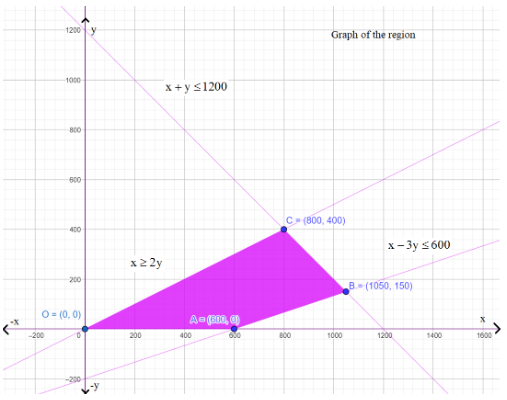Feasible Region having points A (600, 0), B (1050, 150), and C (800, 400) at the corners

The points at the corners in the feasible region areA (600, 0), B (1050, 150), and C (800, 400). The values of Z at these corner points are as follows.

 Corner Point z = 12x + 16y A(600, 0) 7200 B(1050, 150) 15000 C(800, 400) 16000 $\rightarrow maximum$

Thus, the maximum value of z is 16000 at point (800, 400).

### An Overview of NCERT Solutions for Class 12 Maths

Most of the time, students face a bit of difficulty in finding the right CBSE NCERT Solutions for Class 12 Maths Chapter 12 Linear Programming. In such cases, students access the solutions of Linear Programming Class 12 that are prepared by the professionals who have years of teaching experience. As these solutions are available online, students can download this file at any given point in time and go through it completely. It will help them achieve a good score on the exam and shape their future.

### What is Linear Programming?

Linear programming is a method to optimise any problem with several variables and constraints. It is a simple but powerful tool every data scientist should be aware of. The linear programming method is used to solve optimisation problems where all the constraints, along with the objective function, are linear equalities or inequalities

### Topics Covered Under the Chapter 12-Linear Programming

• Introduction to Linear Programming

• Linear Programming Problem and its Mathematical Formulation

• Mathematical Formulation of the Problem

• Graphical Method of Solving Linear Programming Problems

• Different Types of Linear Programming Problems

Now, let us discuss each topic in detail.

#### 12.1 Introduction

In NCERT Class 12 Maths Chapter 12 will introduce you to Linear Programming. In earlier classes, you have been introduced to and taught linear equations. You have also studied linear inequalities and systems of linear equations in two variables and how to find their solutions by graphical method. In this chapter, you will learn how to plot linear equations on a graph. Since many applications in Mathematics involve systems of inequalities or equations, this chapter will teach you how to apply these inequalities or equations. You will be provided with an example in the Chapter 12 Maths Class 12 NCERT Solutions to understand this chapter more. You will be given a situational example and taught what optimization problems are.

#### 12.2 Linear Programming Problem and its Mathematical Formulation

In this LPP Class 12, you will be taught further about the mathematical formulation of the problem in two variables from the same example in the previous section. You will get to learn how to solve and understand the mathematical formulation of the problem. You will understand the significance of non-negative constraints and their role in linear programming. You will learn the use and importance of certain terms used in linear programming problems, such as objective function, constraints, and optimization problems.

In this Chapter 12 Maths Class 12, you will learn the graphical method of solving linear programming problems. You will learn how a graph consists of common to all half-planes, which is determined by the inequalities. You will also study in Chapter 12 Maths Class 12 that the graphs to plot inequalities or equations have terms that come handy while solving a linear programming problem such as feasible choice, feasible region, and feasible solutions. You will learn why any point outside the feasible region is called an infeasible solution. Then there is another point that you will study as an optimal (feasible) solution. You will also learn two important theorems that will enable a better understanding of the inequalities following some examples to help you understand the theorems.

### Exercise 12.1 – 10 Questions (All Questions Require Graphs and Long Questions)

#### 12.3 Different Types of Linear Programming Problems

In the linear programming Class 12 solutions, you will learn about some different types of linear programming problems. For example, manufacturing problems where you will learn how to utilise this method to keep track and make maximum profit. In Class 12 Maths Chapter 12 NCERT Solutions, you will learn how to utilise this method to keep track and control of constituents and nutrients. Finally, there is a transportation problem where you will learn how to keep track and schedule of transportation. Following this in LPP solutions Class 12, you will be given examples that will help you understand these methods.

### Key Features of NCERT Solutions for Class 12 Maths Chapter 3

It is required to go through every segment of this chapter to increase your knowledge and perception of the subject thoroughly. When you go through LPP Class 12 NCERT solutions that are created by the proficient professionals in this subject, you will get an unfluctuating understanding of the chapter and learn the elementary and advanced concepts and theorems. The key features are listed below:

• You will most definitely attain more knowledge about the concepts and theorems mentioned in NCERT Solutions for Class 12 Maths Chapter 12.

• You will learn how to assess your knowledge gap with the help of the linear programming Class 12 solutions PDF.

• You learn how to upgrade your knowledge in the main sections.

• You will be able to understand your assignments based on concepts related to linear programming Class 12 Chapter 12.

## Importance of CBSE Class 12 Maths Chapter 12 Linear Programming NCERT Solutions

Class 12 Maths Chapter 12 is a crucial part of the syllabus. It ensures the development of concepts related to linear programming. It is an important topic used in different subjects and various aspects of life. Hence, the preparation of this chapter becomes mandatory for the students of Class 12.

To prepare this chapter well, students will need the complete study material to get a simpler explanation of the concepts. Along with these notes, they will also need the assistance of exercise solutions for this chapter. Once they have completed studying all the concepts and principles, they will need these solutions to practise solving the exercise questions.

The guidance offered by the NCERT solutions for Class 12 Maths Chapter 12 Linear Programming will help students proceed with their preparation faster. The answers compiled by the experts follow the latest CBSE syllabus and guidelines. Hence, practising solving the exercise questions by following these solutions will make it easier to answer such questions perfectly and focus on the development of a strong conceptual foundation. This is why the solutions to these exercises are part and parcel of the study material for Class 12 Maths Chapter 12.

## Advantages of CBSE Class 12 Maths Chapter 12 Linear Programming NCERT Solutions

• Based on the common issues students face while solving these exercise problems, the solutions have been compiled. The answers to the fundamental questions have been kept in a simpler version for easier comprehension of concepts and answer formats.

• The solutions can be accessed online or offline when you download the file on your computer. You can easily check these solutions to resolve doubts at your convenience and take your preparation ahead.

• Focus on how the critical questions have been answered by using the concepts and formulas taught in this chapter. Follow the same to develop your answering skills and score more in the exams.

## Key Features of NCERT Solutions for Class 12 Maths Chapter 3

It is required to go through every segment of this chapter to increase your knowledge and perception of the subject thoroughly. When you go through LPP Class 12 NCERT solutions that are created by proficient professionals in this subject, you will get an unfluctuating understanding of the chapter and learn the elementary and advanced concepts and theorems. The key features are listed below:

• Comprehensive explanations for each exercise and questions, promote a deeper understanding of the subject Maths.

• Clear and structured presentation for easy comprehension.

• Accurate answers aligned with the curriculum, boosting students' confidence in their knowledge.

• Visual aids like diagrams and illustrations to simplify complex concepts.

• Additional tips and insights to enhance students' performance.

• Chapter summaries for quick revision.

## Download CBSE Class 12 Maths Chapter 12 Linear Programming NCERT Solutions PDF

Get the free PDF version of these solutions for this chapter and complete your study material. Focus on how to use these solutions when practising solving exercise questions. Learn to keep your answers to the point and use the exam time more efficiently. Develop your knowledge and skills to stay ahead of the competition.

## Conclusion

Exploring Vedantu's NCERT Solutions for Class 12 Maths Chapter 12 on Linear Programming provides a valuable learning experience. The clear explanations and step-by-step solutions make complex concepts more accessible. With Vedantu, students can grasp the difficulties of linear programming effortlessly. Vedantu's commitment to quality education is evident in our user-friendly approach, making math learning enjoyable. By utilizing these NCERT Solutions, students not only enhance their problem-solving skills but also gain confidence in tackling mathematical challenges. Vedantu's NCERT Solutions serve as a reliable companion for Class 12 students, fostering a deeper understanding of linear programming in a simple and effective manner.

## FAQs on NCERT Solutions for Class 12 Maths Chapter 12 - Linear Programming

1. How many sums are there in the NCERT Solutions for Class 12 Maths Chapter 12- Linear Programming?

There are 3 exercises in the NCERT Class 12 Maths Chapter 12. In the first exercise, Ex.-12.1, there are 10 sums. In the second exercise, Ex.-12.2, there are 11 sums, and in the miscellaneous exercise, there are 10 sums. All the sums in this chapter are solved with stepwise explanations in the NCERT Solutions for Class 12 Maths Chapter 12- Linear Programming.

2. What is meant by Linear Programming in mathematics?

In linear programming, mathematical functions are subjected to various constraints and thereby maximised or minimised accordingly. All the constraints and the objective functions dealt with in this chapter are linear. The maximum and minimum values of the linear functions are plotted on the xy coordinate for further deductions. The main purpose of linear programming is to optimise various mathematical functions in terms of linear constraints.

3. Can I get NCERT Solutions for Class 12 Maths Chapter 12- Linear Programming online?

Yes, you can get the NCERT Solutions for Class 12 Maths Chapter 12- Linear Programming on Vedantu. The solutions to all the sums are prepared by our subject matter experts for the reference of students. You can download and refer to the NCERT solutions to get a clear understanding of the best problem-solving techniques for the sums of linear programming. The stepwise solutions facilitate a self-study session as you can compare your answers with these expert solutions.

Yes, you can download the NCERT Solutions for Class 12 Maths Chapter 12- Linear Programming for free from Vedantu. These NCERT Solutions are available in a PDF format and you can download the same from our mobile application as well. This feature of free downloadable NCERT Solutions of Vedantu enables all students with an internet connection to refer to the best study materials online.

5. What are the fundamental theorems for linear programming?

Theorem 1

Let us consider  R as the feasible region for linear programming and let's consider z to be ax+by, which is the objective function. When z reaches an optimal value, whether it be maximum or minimum and the variables x and y are the subjects to constraints. This optimal value that occurs must occur at a corner point of the said feasible region.

Theorem 2

Let us consider  R as the feasible region for the given linear programming problem and let z be ax+by, which is the objective function. When the R is bounded then z attains both the maximum and the minimum value of R where each recurs at the joint corner of JR.

6. What are the steps followed to apply the corner point method?

The steps used in applying the corner point method are as follows:

• First, it is important to find the feasible regions in the given linear programming problem, and along with it determine the corner point. This can be done by inspection or by solving the two equations of the line that intersect at that point.

• The next step evolves the evaluation of the objective function, ie, z= ax+by at each of the corner points. Denote M and m respectively as the largest and smallest values of the given points.

• When the feasible regions are bounded, then M and m respectively attain the maximum and the minimum of the objective function at the corner points.

7. What is a feasible region?

A feasible region attributed to the common region is determined by all the constraints which include even the non-negative constraints x,y>0 of linear programming. All other regions that do not fall under the feasible regions are termed as the non-feasible regions.

8. What is an objective function and a constraint?

An objective function refers to the linear function, for instance, z=px+py, where p and q are the constants. This linear equation that needs to be maximised or minimised is referred to as the objective function. When restrictions or linear inequalities are placed on a variable in the linear programming problem it is known as constraints. It is important for the student to familiarise and be thorough with these concepts in order to be able to do well in the examination.

9. How to prepare for Class 12 Maths Chapter 12?

A subject such as Math demands a high amount of practice to be thorough with the concepts. With adequate and regular practice, the student will have all the important points at the tip of their fingers.  Therefore, without any doubt, the student needs to practise all the exercises that this chapter entails. To add the icing to the cake, the students can turn towards the NCERT Solutions for Class 12 Maths Chapter 12 available on the Vedantu website and on the Vedantu app at free of cost. These solutions will prove to be the best source of guidance for the students making them exam ready.